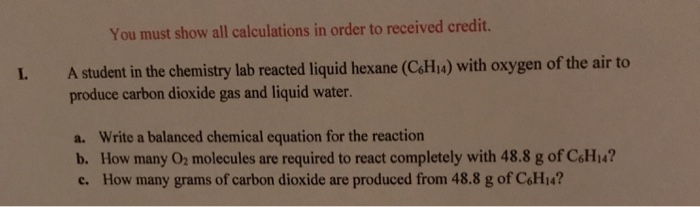Question

# Which one of the following is an example of a balanced chemical reaction? C3H6O + 4O2...

Which one of the following is an example of a balanced chemical reaction? C3H6O + 4O2 → 3CO2 + 3H2O

2C3H6O + 9O2 → 6CO2 + 6H2O

C3H6O + 3O2 → 3CO2 + 3H2O

2C3H6O + 9O2 → 6CO2 + 3H2O

C3H6O + 9O2 → 3CO2 + 3H2O

balanced chemical reaction is C3H6O + 4O2 → 3CO2 + 3H2O

First of all balance the carbon and hydrogen as per number of hydrogens and carbons are present in the reactant C3H6O.

After that balance the oxygen on both sides(first counte the oxygen atoms on product side and then put desired stoichiometric coefficient of O2 on the reactant side).

#### Earn Coins

Coins can be redeemed for fabulous gifts.

Similar Homework Help Questions
• ### Consider the following equation for the combustion of acetone (C3H6O)(C3H6O), the main ingredient in nail polish...

Consider the following equation for the combustion of acetone (C3H6O)(C3H6O), the main ingredient in nail polish remover. C3H6O(l)+4O2(g)→3CO2(g)+3H2O(g)C3H6O(l)+4O2(g)→3CO2(g)+3H2O(g) ΔHrxn=−1790kJ If a bottle of nail polish remover contains 154 gg of acetone, how much heat would be released by its complete combustion?

• ### Consider the following thermochemical equation for the combustion of acetone (C3H6O), the main ingredient in nail...

Consider the following thermochemical equation for the combustion of acetone (C3H6O), the main ingredient in nail polish remover. C3H6O(l)+4O2(g)→3CO2(g)+3H2O(g)ΔH∘rxn=−1790kJ If a bottle of nail polish remover contains 174 mL of acetone, how much heat would be released by its complete combustion? The density of acetone is 0.788 g/mL.

• ### Consider the following thermochemical equation for the combustion of acetone, C3H6O, the main ingredient in nail...

Consider the following thermochemical equation for the combustion of acetone, C3H6O, the main ingredient in nail polish remover: C3H6O(l)+4O2(g)→3CO2(g)+3H2O(g) ΔH∘rxn=−1790kJ If a bottle of nail polish remover contains 173 mL of acetone, how much heat would be released by its complete combustion? The density of acetone is 0.788 g/mL. Express your answer in kilojoules.

• ### Combustion of the reaction for one mole of liquid Acetone DeltarH= -1755 kj/mol DeltarU=-1753 kj/mol C3H6O...

Combustion of the reaction for one mole of liquid Acetone DeltarH= -1755 kj/mol DeltarU=-1753 kj/mol C3H6O + 4O2 (g) -> 3CO2 (g) + 3H2O a) If you were using a bomb calorimeter , what mass of the Water could be heated form 21.0 celsius to 61.0 celsius by burning 0.6g of Acetone in the calorimeter. The specific heat of the Water is 4.184 j/g c b) What would the heat capacity of the calorimeter as whole if the burning of...

• ### For the balanced equation, I got: C6H12+9O2=6CO2+6H2O...is this correct? A student in the chemistry lab reacted...For the balanced equation, I got: C6H12+9O2=6CO2+6H2O...is this correct? A student in the chemistry lab reacted liquid hexane (C_6H_14) with oxygen of the air to produce carbon dioxide gas and liquid water. Write a balanced chemical equation for the reaction How many O_2 molecules are required to react completely with 48.8 g of C_6H_14? How many grams of carbon dioxide are produced from 48.8 g of C_6H_14?

• ### Which of the following is true about the following chemical reaction at equilibrium? PICK ALL THAT...

Which of the following is true about the following chemical reaction at equilibrium? PICK ALL THAT APPLY. C6H12O6 + 6O2 6CO2 + 6H2O + Energy A. The concentration of H2O equals the concentration of O2. B. The rate of the forward reaction, reactants to products, equals the rate of the reverse reaction, products to reactants. C. The C-C bonds hold more energy in C6H12O6 than the C-O bonds in CO2. Hint: think teter-toter. D. The concentration of O2 is changing...

• ### which one of the following is the balanced chemical equation for the reaction of iodide with...

which one of the following is the balanced chemical equation for the reaction of iodide with peroxydisulfate and what type of reaction is this? a) I2+2S2O32--->2I-+ S4O62- b) 2I-+S4O62--->I2 + 2S2O32- c) S2O82-+2I- --> I2 +SO42- d) I2- +2SO42---> 2S2O82- + 2I- e) 2I- + 2SO42- --> I2+ 2S2O82- acid base redox neutralization precipitation metathesis

• ### Give an example of a redox reaction. Include the balanced chemical equation.

Give an example of a redox reaction. Include the balanced chemical equation.

• ### 8. Given the following balanced reaction for the combustion of ethanol, an alternative fuel and gasoline...

8. Given the following balanced reaction for the combustion of ethanol, an alternative fuel and gasoline additive C2H5OH(l) + 3O2(g) → 2CO2(g) + 3H2O(g) and the following table of standard enthalpies of formation. How much energy (in kJ) is released from the combustion of 1.25kg of ethanol? (Continue on the next page)

• ### Which of the following chemical equations is balanced? Fe2O3 + CO → Fe + 2CO2 Na2SO2...

Which of the following chemical equations is balanced? Fe2O3 + CO → Fe + 2CO2 Na2SO2 + S → S2O3 + S 3CuO + 2NH3 → 3Cu + 3H2O + N2 4Al + 3Fe3O4 → 4Al2O3 + 9Fe